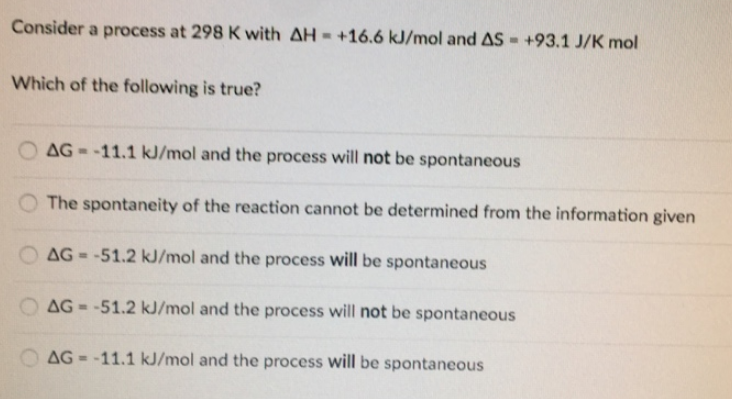# Problem: Consider a process at 298 K with ΔH = +16.6 kJ/mol and ΔS = +93.1 J/K mol Which of the following is true? • ΔG = -11.1kJ/mol and the process will not be spontaneous • The spontaneity of the reaction cannot be determined from the information given • ΔG = -51.2 kJ/mol and the process will be spontaneous • ΔG = -51.2 kJ/mol and the process will not be spontaneous • ΔG = -11.1 kJ/mol and the process will be spontaneous

###### FREE Expert Solution
82% (327 ratings)###### Problem Details

Consider a process at 298 K with ΔH = +16.6 kJ/mol and ΔS = +93.1 J/K mol

Which of the following is true?

• ΔG = -11.1kJ/mol and the process will not be spontaneous

• The spontaneity of the reaction cannot be determined from the information given

• ΔG = -51.2 kJ/mol and the process will be spontaneous

• ΔG = -51.2 kJ/mol and the process will not be spontaneous

• ΔG = -11.1 kJ/mol and the process will be spontaneous